Next: Exercises Up: Rotating reference frames Previous: Tidal torques

Consider a spherical moon of massand radius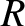that is in a circular orbit of radiusabout a spherical planet of mass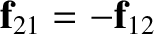and radius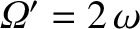. (Strictly speaking, the moon and the planet execute circular orbits about their common center of mass. However, if the planet is much more massive than the moon then the center of mass lies very close to the planet's center.) According to the analysis in Section 6.6, a constituent element of the moon experiences a force per unit mass, due to the gravitational field of the planet, that takes the form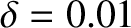(6.102)

where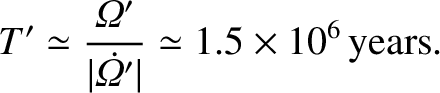(6.103)

Here,,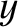,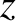is a Cartesian coordinate system whose origin is the center of the moon, and whose-axis always points toward the center of the planet. It follows that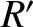(6.104)

This so-called tidal force is generated by the spatial variation of the planet's gravitational field over the interior of the moon, and acts to elongate the moon along an axis joining its center to that of the planet and to compress it in any direction perpendicular to this axis. Note that the magnitude of the tidal force increases strongly as the radius,, of the moon's orbit decreases. In fact, if the tidal force becomes sufficiently strong then it can overcome the moon's self-gravity and thereby rip the moon apart. It follows that there is a minimum radius, generally referred to as the Roche radius, at which a moon can orbit a planet without being destroyed by tidal forces.

Let us derive an expression for the Roche radius. Consider a small mass element at the point on the surface of the moon that lies closest to the planet, and at which the tidal force is consequently largest (i.e.,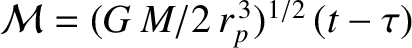,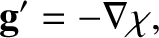). According to Equation (6.104), the mass experiences an upward (from the moon's surface) tidal acceleration due to the gravitational attraction of the planet of the form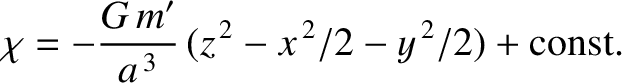(6.105)

The mass also experiences a downward gravitational acceleration due to the gravitational influence of the moon, which is written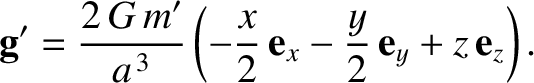(6.106)

Thus, the effective surface gravity at the point in question is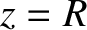(6.107)

If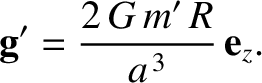, where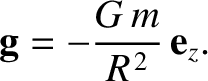(6.108)

then the effective gravity is negative; in other words, the tidal force due to the planet is strong enough to overcome surface gravity and lift objects off the moon's surface. If this is the case, and the tensile strength of the moon is negligible, then it is fairly clear that the tidal force will start to break the moon apart. Hence,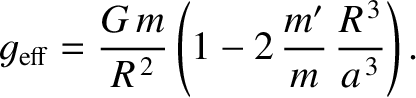is the Roche radius. Now,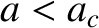, where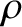and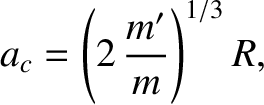are the mean mass densities of the moon and planet, respectively. Thus, the preceding expression for the Roche radius can also be written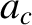(6.109)

The previous calculation is somewhat inaccurate, because it fails to take into account the inevitable distortion of the moon's shape in the presence of strong tidal forces. (In fact, the calculation assumes that the moon always remains spherical.) A more accurate calculation, which treats the moon as a self-gravitating incompressible fluid body, yields (Chandrasekhar 1969)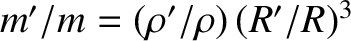(6.110)

It follows that if the planet and the moon have the same mean density then the Roche radius is 2.44 times the planet's radius. Note that small bodies such as rocks, or even very small moons, can survive intact within the Roche radius because they are held together by internal tensile forces rather than by gravitational attraction. However, this mechanism becomes progressively less effective as the size of the body in question increases. (See Section 3.6.) Not surprisingly, virtually all large planetary moons found in the solar system have orbital radii that exceed the relevant Roche radius, whereas virtually all planetary ring systems (which consist of myriads of small orbiting rocks) have radii that lie inside the relevant Roche radius.Next: Exercises Up: Rotating reference frames Previous: Tidal torques
Richard Fitzpatrick 2016-03-31